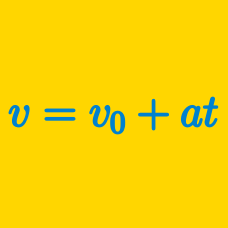Classical Mechanics

# Velocity vs. Acceleration

At time zero, a green car and a red car start from rest on a straight road from the same position in adjacent lanes. The green car moves with a constant speed of $21 \text{ m/s}$ and the red car accelerates uniformly at a rate of $1.5 \text{ m/s}^2 .$ Which car is ahead $10$ seconds later?

A car initially at rest starts to travel on a straight road. The car starts moving with a constant acceleration of $12 \text{ m/s}^2.$ After accelerating for $4$ seconds, the driver applies the brakes, and the car decelerates at a constant rate of $-6 \text{ m/s}^2 .$ From the point the car's brakes are applied, how long does it take for the car to come to a complete stop?

A body rises vertically up to a height of $45 \text{ m}$ in $3$ seconds, then falls back to the point of projection, with a constant acceleration during its entire movement. Assuming that the air resistance is negligible, is the following statement true or false?

"During its entire movement, the acceleration of the body is $6 \text{ m/s}^2,$ and its average velocity is $25 \text{ m/s}.$"

Cars $A$ and $B$ are moving in the same direction in adjacent straight lanes. If car $A$ has a bigger acceleration than car $B,$ does it mean that car $A$ is faster than car $B?$

Something that is accelerating is $\text{\_\_\_\_\_\_\_\_\_}.$

×